ggplot2 offers the fantastic option for displaying complex data in forms of 'many multiple', i.e. the facets. From the example of `facet_grid`:

``````p <- ggplot(mpg, aes(displ, cty)) + geom_point()
p + facet_grid(drv ~ cyl) + theme_bw()
``````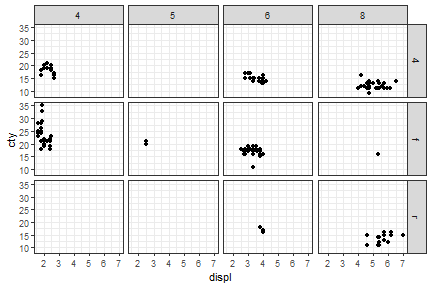In the above example, the panel's borders are drawn with the default settings of `theme_bw()`.

If we desire the the axis lines, such as those given in this package, the distinction of the panels disappears.

``````library(lemon)
p <- p + coord_capped_cart(bottom='both', left='both') +
theme_bw() + theme(panel.border=element_blank(), axis.line=element_line())
p + facet_grid(drv ~ cyl)
``````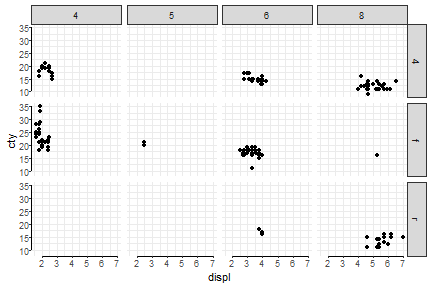The above example is re-created below with both left- and bottom-axis lines repeated.

``````library(lemon)
p + facet_rep_grid(drv ~ cyl) + coord_capped_cart(bottom='both', left='both') +
theme_bw() + theme(panel.border=element_blank(), axis.line=element_line())
``````
``````## Coordinate system already present. Adding new coordinate system, which will replace the existing one.
``````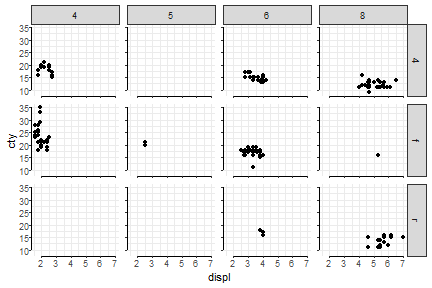## Keeping (some) labels

In the following example, we change the facet from a grid to being wrapped on the interaction of `drv` and `cyl`, and add free scaling on y-axis. `facet_wrap` would normally print the y-axis tick labels for each panel, but still ignores the x-axis.

``````p + facet_wrap(~ interaction(cyl, drv), scales='free_y')
``````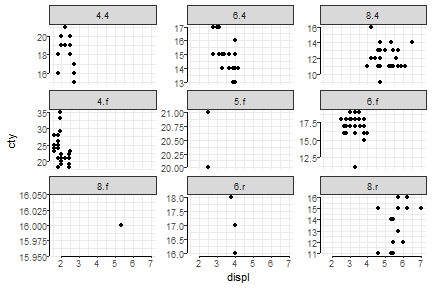A work around by keeping both axes free scale, and fixing the x-axis with either `scale_x_continuous` or limits in `cord_*`, but the same x-axis tick labels are repeated. And this is a bit tedious.

``````p + facet_wrap(~ interaction(cyl, drv), scales='free') +
coord_capped_cart(bottom='both', left='both', xlim=c(2,7))
``````
``````## Coordinate system already present. Adding new coordinate system, which will replace the existing one.
``````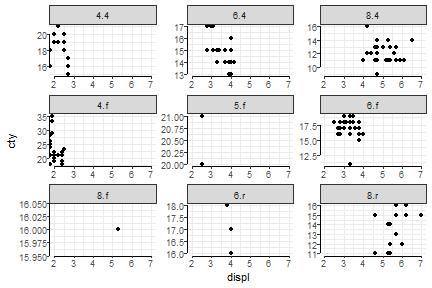We can specify which labels to keep with `facet_rep_wrap`. Default is `repeat.tick.labels=FALSE` when `scales='fixed'` which removes tick labels on all axes (shown in earlier figure). When using free scales on `facet_rep_wrap`, the appropiate labels are drawn.

``````p + facet_rep_wrap(~ interaction(cyl, drv), scales='free_y', repeat.tick.labels = 'left')
``````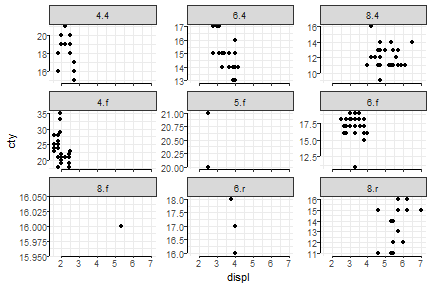The argument `repeat.tick.labels` accepts a range of arguments: `top`,`right`,`bottom`, and `left`, any combination of these are accepted, as well as `TRUE` and `FALSE` which are interpreted as all or none, and `all` and `none`.

## Examples

There are many posibilities. Examples given below (but not executed), and they might not be pretty.

``````p + facet_rep_wrap(~ interaction(cyl, drv), scales='free_y', repeat.tick.labels = 'all')
p + facet_rep_wrap(~ interaction(cyl, drv), scales='free_y', repeat.tick.labels = 'bottom')
p + facet_rep_wrap(~ interaction(cyl, drv), scales='free_y', repeat.tick.labels = 'left')
p + scale_x_continuous(sec.axis = dup_axis()) +
facet_rep_wrap(~ interaction(cyl, drv), scales='free_y', repeat.tick.labels = 'all')
p + scale_x_continuous(sec.axis = dup_axis()) +
facet_rep_wrap(~ interaction(cyl, drv), scales='free_y', repeat.tick.labels = c('top','left'))
``````